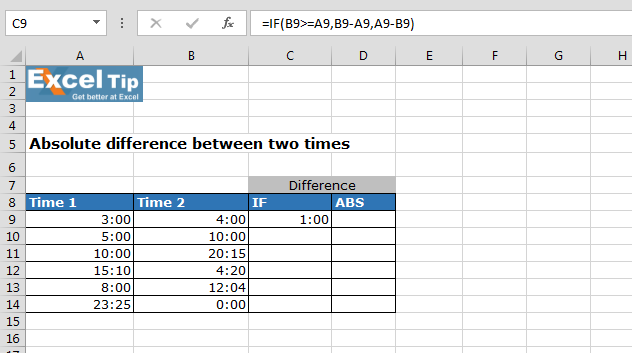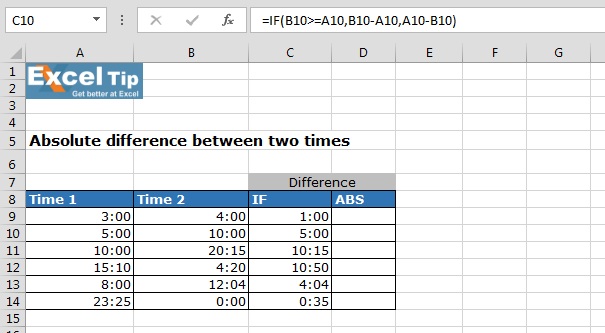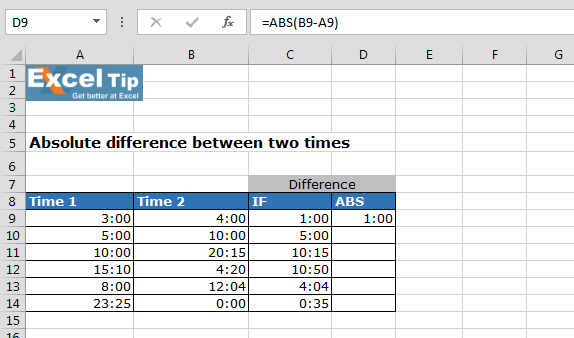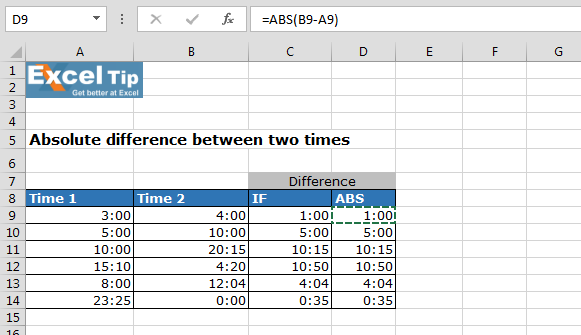# Calculating Absolute Difference between Two Time Values

In this article, you’ll learn how to calculate absolute difference between two time values in Microsoft Excel. In Excel, we have several ways to calculate the absolute difference, but we will learn by using IF function and ABS function.

Let’s take an example,

We have 2 time range in Column A and, in Column B, we want to calculate the absolute difference between both times.• Enter the formula in cell C9
• =IF(B9>=A9,B9-A9,A9-B9)
• Press Enter• The function will return 1:00 which means difference between both times is 1 hour
• To get the result for all, copy the formula from cell B9 to range B10:B14Formula Explanation:-=IF(B10>=A10,B10-A10,A10-B10): in this formula, we have defined that if time of cell B10 is less than and equals to the time of cell A10, then cell B10 time will be subtracted from the time of cell A10 else cell A10 time will be subtracted from the cell B10 time.

Now, How to use ABS function to calculate the difference between two time values?

Follow below given steps and formula:-

• Enter the formula in D9
• =ABS(B9-A9)
• Press Enter
• The function will return 1:00 which means 1 hour difference is here• Copy the same formula in the range D10:D14Note: - After applying formula format cell as hh:mm.

In this way, we can calculate the absolute time difference in Microsoft Excel.